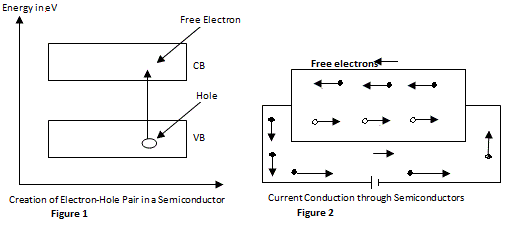# Intrinsic and Extrinsic Semiconductors in Basic Electronics

INTRODUCTION :

Different materials have different properties we shall consider the property of conduction of electricity, by any material. Certain materials like copper, silver and gold are good conductors of electricity; whereas materials like glass, wood, paper, etc., are bad conductors or insulators for electricity. Both the conductors and the insulators find wide applications in engineering industry.

There is another category of material whose conductivity lies in between conductors and insulators. Such materials are called semi conductors. Germanium and silicon are two of the well- known semi conductor materials. Further, in this chapter, we shall study the intrinsic and extrinsic semiconductors, PN junction diode zener diode and transistor operation and characteristics.

CONDUCTORS, INSULATORS & SEMICONDUCTORS :

The electrical properties of different materials can be explained in terms of the electrons having energies in the valence band and conduction band, because the electrons lying in the lower energy bands which are normally filled, play no role in the conduction process.

(i) Insulators : Insulators are the solid materials which are bad conductors of electricity. ex : Wood, Plastic, rubber. In terms of energy bands, Insulators

(a) have full valence band.
(b) have an empty conduction band
(c) have a wide forbidden energy gap.

So in case of insulators a very large amount of energy must be supplied to cause an electron to cross from the valence band to the conduction band.

(ii) Conductors: Conductors are the solid materials which are good conductors of electricity. ex: Copper, Aluminium, gold. In terms of energy bands conductors have –

(a) No forbidden energy gap between valence band and conduction band i.e., Valence band and conduction band are overlapped.
So in case of conductors very large number of free electrons are available even at extremely low temperatures.

(iii) Semi Conductors : Semi conductors are the solid materials which are neither good conductors nor bad conductors of electricity. ex : Silicon, Germanium.
In terms of energy band diagrams semiconductors have
(i) Narrow forbidden energy gap EG 1eV.
(ii) Partially filled valance and conduction bands at room temperature.

INTRINSIC AND EXTRINSIC SEMICONDUCTORS:

Semiconductors can be classified into two types : (1) Intrinsic semiconductors and (2) Extrinsic semiconductors.

1. Intrinsic Semiconductors :

A semiconductor in an extremely pureform of state is known as intrinsic semiconductor. Even at room temperature, some of the valence electrons acquire sufficient energy to enter the CB. To form free electrons. Under the influence of the electric field, these electrons contribute to electric current.
A missing electron in the VB leaves a vacant space there, called a hole . Holes also contribute to electric current.Creation and Conduction through Semiconductor
In an intrinsic semiconductors, event at room temperature, electron-hole pairs are created. When electric field is applied in an intrinsic semiconductor, the current conduction occurs by two courses, specifically by free electrons and holes. Under the influence of electric field, conduction through the semiconductor is both by free electrons and holes. The band gap energy for Ge is 0.7 eV and Si is 1.1 eV.

2. Extrinsic Semiconductors:

In order to increase the conductivity of a semiconductor, an impurity is added to it and this process is called doping, The impurity called doping material or dopant.

The resultant material after doping is called extrinsic semiconductor. The amount of impurity added is extremely small, say 1 or 2 impurity atoms for 108 atoms of pure semiconductors.

The usual dopants are :

(i) Pentavalent atoms having five valence electrons. Arsenic (As) Antimony (Sb), phosphorus (P).

(ii) Trivalent atoms having three valence electrons.   gallium (Ga), indium (In), aluminium (Al), boron (B).

Pentavalent impurities are called donor impurities because they donate free electrons to the semiconductor.

Trivalent impurities are called acceptor impurities because the holes created can accept the electrons.

Based on the kind of impurity added, extrinsic semiconductors are divided into :
(i) N- type semiconductor and (ii) P- type semiconductor.

DIFFERENCES BETWEEN INTRINSIC AND EXTRINSIC SEMICONDUCTORS

 No: Intrinsic Semiconductor Extrinsic Semiconductor 1 It is a pure semiconductor. It could be either Ge or Si These are obtained by adding impurities to pure semiconductor. These may be p-type or n-type. 2 At zero temperature, it becomes insulator. Such is not a case here. 3 Conductivity increases with temperature. Conduction is possible at room temperature also. 4 Conductivity is in between metals and insulators; it is less compared to extrinsic semiconductors. Conductivity is much higher, than intrinsic semiconductors (but less than metals). 5 No. of electrons and holes are equal. No. of electrons and holes are not equal. In n-type, electrons > > holes. In p-type, holes > > electron 6 Fermi level is exactly midway between conduction band and valence band. Fermi level is close to conduction band in n-type and is close to valence band in p-type.